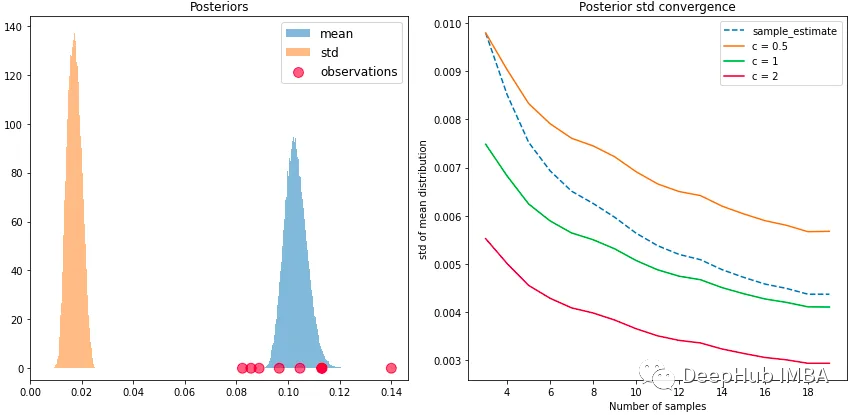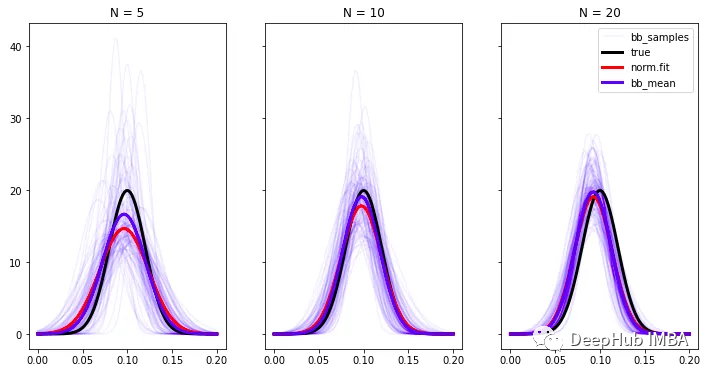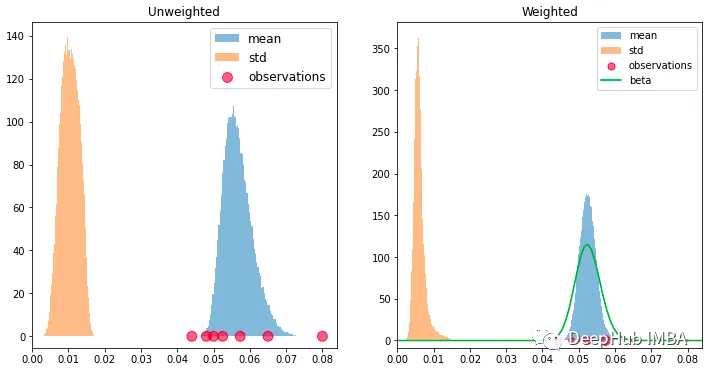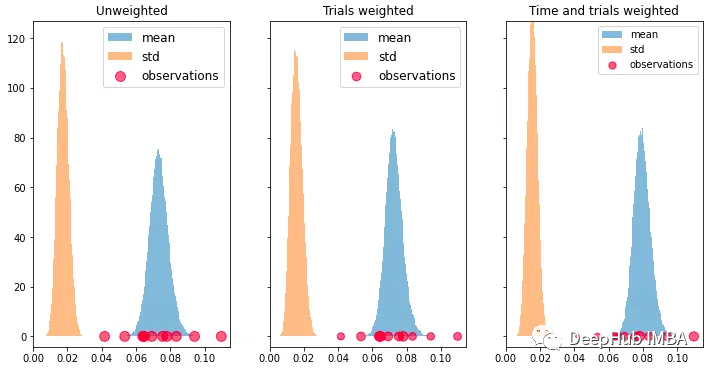0

# 带加权的贝叶斯自举法 Weighted Bayesian Bootstrap

## Python实现

`````` importnumpyasnp
importscipy.statsasss

classBayesianBootstrap:

def__init__(self, concentration: float=1.):
self.n_draws=100000
self.bins=100
self.concentration=concentration

defsample(self, obs: np.ndarray, weights: np.ndarray=None):

# If no weights passed, use uniform Dirichlet
ifweightsisNone:
weights=np.ones(len(obs))

# Normalize weights to mean concentration
weights=weights/weights.mean() *self.concentration

# Sample posteriors
draws=ss.dirichlet(weights).rvs(self.n_draws)
means= (draws*obs).sum(axis=1)
vars=draws* (obs-means.reshape(self.n_draws, 1)) **2

returnmeans, np.sqrt(vars.sum(axis=1))

defdistribution(self, obs: np.ndarray, weights: np.ndarray=None):

# Sample and create distribution objects
means, stds=self.sample(obs, weights)
hist_mean=np.histogram(means, bins=self.bins)
hist_std=np.histogram(stds, bins=self.bins)

returnss.rv_histogram(hist_mean), ss.rv_histogram(hist_std)
``````

.sample()方法是这里的核心，该方法计算来自观察的后验样本的平均值和标准偏差。与经典的自举法相比即使对于非常大量的样本，因为使用了向量化的计算，所以不必担心速度问题。distribution()函数是从样本直方图中返回scipy分布对象，这样我们可以使用标准的API来计算任何后验统计信息。## 分布估计## 加权

`````` rate_true=0.05
trials=np.array([50, 100, 200, 500, 800, 1000, 1500])
success=ss.binom.rvs(trials, rate_true)
obs=success/trials
```````````` x=np.linspace(0, 0.1, 100)
a=success.sum()
b=trials.sum() -a
beta_pdf=ss.beta(a, b).pdf(x)
``````

`````` rates_true=np.linspace(0.05, 0.1, size)
trials_mean=np.linspace(1000, 1200, size)
trials=ss.norm(trials_mean, 200).rvs(10).astype(int)
success=ss.binom.rvs(trials, rates_true)

obs=success/trials
time_weights=np.log2(np.linspace(1.1, 2, size))
weights=trials*time_weights
``````## 总结

• 只有很少的数据
• 关心的是估计的不确定性
• 要尽可能地保持公正

### “带加权的贝叶斯自举法 Weighted Bayesian Bootstrap”的评论:

##### 关于作者##### Deephub